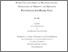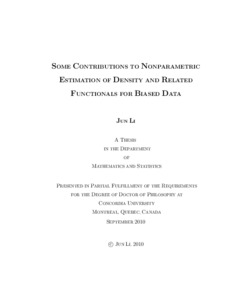Title:

Some Contributions to Nonparametric Estimation of Density and Related Functionals for Biased Data

Li, Jun (2010) Some Contributions to Nonparametric Estimation of Density and Related Functionals for Biased Data. PhD thesis, Concordia University.Preview
Text (application/pdf)
thesis.pdf - Accepted Version
1MB

Abstract

Length biased sampling, as a special case of general biased sampling, occurs naturally in many statistical applications. In problems related with such applications, two different density functions are involved. One of them is the density of interest, which is referred to as the unweighted density, information about which is not observable directly in practice; the other one is referred to as the weighted density, the sample from which could be observed directly. These two densities are connected through a weight function. One aspect regarding data from weighted density is to estimate the unweighted density from the sample obtained using the weighted density. In this thesis we concentrate on the weight function representing length of the sampling unit that results in a sample called length-biased sample. Since most of such data are nonnegative, unweighted density has a non-negative support where common kernel density estimators with symmetric kernel may not be appropriate. Such density estimators usually generate the edge effect, which makes these to have large bias at the lower boundary. One possible reason for this is that symmetric kernels may assign some weights in region of zero probability.

In this thesis, we propose some new smooth density estimators based on Poisson distribution and nonnegative asymmetric kernels for length biased data to take care of the edge effect. We investigate asymptotic behavior of these proposed density estimators as well as their finite sample performance through extensive simulation studies, that is more meaningful in practice. Also, we compare our new density estimators with other estimators in literature. Further, in addition to density estimators, we also consider smooth estimators of distribution function and some other functionals of the density such as hazard function and mean residual life function.

Divisions: Concordia University > Faculty of Arts and Science > Mathematics and Statistics Thesis (PhD) Li, Jun Concordia University ﻿Ph. D. Mathematics 7 December 2010 Chaubey, Yogendra P. 6980 JUN LI 13 Jun 2011 13:58 18 Jan 2018 17:29
All items in Spectrum are protected by copyright, with all rights reserved. The use of items is governed by Spectrum's terms of access.

Repository Staff Only: item control page

Back to top